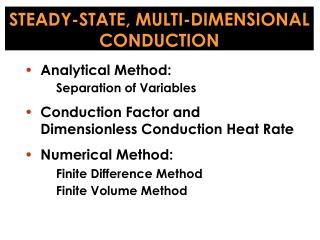Download Presentation# STEADY-STATE, MULTI-DIMENSIONAL CONDUCTION - PowerPoint PPT PresentationDownload Presentation- - - - - - - - - - - - - - - - - - - - - - - - - - - E N D - - - - - - - - - - - - - - - - - - - - - - - - - - -
##### Presentation Transcript

1. STEADY-STATE, MULTI-DIMENSIONAL CONDUCTION • Analytical Method: • Separation of Variables • Conduction Factor and Dimensionless Conduction Heat Rate • Numerical Method: • Finite Difference Method • Finite Volume Method

2. Eigenvalue problem in the direction of homogeneous boundary condition Analytical Method Separation of Variables + Boundary conditions 1) Equation : linear and homogeneous 2) Boundary condition : homogeneous at least in one direction

3. over or boundary conditions : Sturm-Liouville Theory Sturm-Liouville System : Hermitian operator (self-adjoint operator) w(x) : weighting function Corresponding eigenvalue problem

4. boundary terms = boundary terms thus Definition of inner product : formally self-adjoint operator

5. i) ii) when or and can be finite. iii) when either at a or b boundary terms homogeneous boundary conditions

7. : at least piecewise continuous function : eigenfunction Properties of Hermitian operator • eigenvalues : all real • eigenfunctions : orthogonal • eigenfunctions : a complete set orthogonality: : least square convergence

8. Example : Laplace equation y x assume then Ex) 1) in (x,y) coordinate system (Cartesian) with homogeneous boundary conditions in the x direction This is a special case of S-L system with eigenfunctions : trigonometric functions, sinx or cosx

9. r z assume , then or or This is a special case of S-L system with Remark : transform with Bessel equation : Ex) 2) in (r,z) coordinate system (cylindrical) with homogeneous boundary conditions in the r direction eigenfunctions : Bessel functions

10. r q with homogeneous boundary conditions in the q direction assume equation for or with or This is a special case of S-L system with Legendre equation : Remark 3) in (r,q) coordinate system (spherical) eigenfunctions : Legendre functions

11. T2 T1 T1 T1 Let Two dimensional conduction in a thin rectangular plate or a long rectangular rod with no heat generation y W T(x,y) b.c. x 0 L b.c.

12. y 1 Let W 0 q(x,y) 0 x 0 L 0 or Dividing both sides by XY, = constant

13. y 1 W 0 q(x,y) 0 x 0 L 0 boundary conditions

14. For X(x): b.c. To be non-trivial : eigenvalue : eigenfunction

15. For Y(y): b.c. particular solution:

16. Multiply both sides by and integrate fromx = 0 toL

17. Solution: where

18. Td 0 0 0 Td Ta Tb Ta 0 0 Tb 0 0 0 = + + + 0 0 0 Tc 0 Tc -T3(x) T0 T0 T0 0 adiabat 0 0 0 0 0 = 0 0 0 0 = 0 0 + 0 + + T1 0 0 0 adiabat 0 0 0 0 -T3(x) Method of Superposition 1) inhomogeneous boundary condition T = T1 + T2 + T3 + T4 2) inhomogeneous equation T = T1 + T2 = T1+ T3 + T4

19. T(ro,z) = 0 T(r,l) = Tl T(r,0) = 0 or Cylindrical Rod r z ro l boundary conditions r: finite, z:

20. assume

21. T(ro,z) = 0 r T(r,l) = Tl T(r,0) = 0 z ro l = finite Boundary conditions = finite

22. = finite, Let then or thus as = finite: such that For R(r): b.c.

23. eigenfunction: l1ro l2ro l3ro l4ro

24. For Z(z): b.c.

25. Solution: where

26. Conduction Shape Factor and Dimensionless Heat Transfer Rate Conduction Shape Factor S: shape factor S : determined analytically two-dimensional conduction resistance

27. A T1 T2 L T2 T1 Ex) Plane wall Cylindrical wall

28. Conduction shape factors and dimensionless conduction heat rates for selected systems

29. or Dimensionless Heat Transfer Rate Objects at isothermal temperature (T1) embedded with an infinite medium of uniform temperature (T2) Characteristic length Dimensionless conduction heat rate

30. (m,n+1) cell (m-1,n) (m+1,n) (m,n) Dy (m,n-1) Dx Numerical Method Finite Difference Method grid discretization node (nodal point)

31. or Similarly,

32. If we neglect (truncation error) and when

33. (m,n+1) (m-1,n) (m+1,n) (m,n) Dy (m,n-1) Dx Finite Volume Method (Energy Balance Method) Assumption of linear temperature profile

34. Then, Similarly, If

35. Convection Boundary Conditions 1) Side (m,n+1) h, T∞ (m,n) (m-1,n) Dy (m,n-1) Dx If or

36. 2) Corner (m,n) (m-1,n) (m,n-1) Dy Dx If

37. 3) Internal corner (m,n+1) (m-1,n) (m,n) (m+1,n) Dy (m,n-1) Dx If or

38. 1) Analytical method (matrix inversion) → Cramer’s rule Ifn = 10, 3×106operations. Ifn = 25, IBM360 1017years 2) Direct (elimination) method (n < 40) Gauss-Jordan elimination method Augmented matrix of operations

39. : pivot element

40. 3) Gauss-Seidel iteration method (n > 100) diagonal dominance → sufficient condition for convergence convergence criterion

41. Dx = 0.25 m Dy = 0.25 m 1 2 1 3 4 3 5 6 5 7 8 7 Example 4.3 Ts = 500 K Fire clay brick 1 m ×1 m k = 1 W/m.K Ts = 500 K Ts = 500 K Find: Temperature distribution and heat rate per unit length T∞ = 300 K h = 10 W/m2.K Air Nodes at the plane surface with convection: When Dx = Dy,

42. inner nodes: convection nodes: Node 1: Node 3: Node 5: Node 2: Node 4: Node 6: Node 7: Node 8: Ts = 500 K 1 2 1 3 4 3 Ts = 500 K Ts = 500 K 5 6 5 7 8 7 T∞ = 300 K h = 10 W/m2.K

43. Using a standard matrix inversion routine, it is a simple matter to find the inverse of , giving